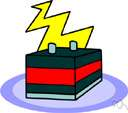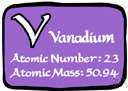# V

Also found in: Thesaurus, Medical, Legal, Financial, Acronyms, Idioms, Encyclopedia, Wikipedia.

## V 1

2. Electricity The symbol for potential difference.
3. also v The symbol for the Roman numeral 5.

abbr.
1. victory
2. vocative
3. volt
4. volume
5. vowel

## v 1

or V  (vē)
n. pl. v's or V's also vs or Vs
1. The 22nd letter of the modern English alphabet.
2. Any of the speech sounds represented by the letter v.
3. The 22nd in a series.
4. Something shaped like the letter V.

abbr.
velocity

(viː) or

## V

n, pl v's, V's or Vs
1. (Linguistics) the 22nd letter and 17th consonant of the modern English alphabet
2. (Phonetics & Phonology) a speech sound represented by this letter, in English usually a voiced labio-dental fricative, as in vote
3.
a. something shaped like a V

## v

symbol for
1. (General Physics) physics velocity
2. (General Physics) specific volume (of a gas)

## V

symbol for
1. (Grammar) (in transformational grammar) verb
2. (General Physics) volume (capacity)
3. (General Physics) volt
4. (Elements & Compounds) chem vanadium
5. (General Physics) luminous efficiency
6. victory
7. (Mathematics) (Roman numeral)five. See Roman numerals
abbreviation for
(Automotive Engineering) Vatican City (international car registration)

## V, v

(vi)

n., pl. Vs V's, vs v's.
1. the 22nd letter of the English alphabet, a consonant.
2. any spoken sound represented by this letter.
3. something shaped like a V.
4. a written or printed representation of the letter V or
v.

## V

1. velocity.
2. verb.
3. victory (by the Allies in WWII).
4. volt.
5. vowel.

## V

Symbol.
1. the 22nd in order or in a series.
2. (sometimes l.c.) the Roman numeral for five. Compare Roman numerals.
4. Biochem. valine.

1. variable.
2. velocity.
3. victory.
4. volt.

## V.

1. valve.
2. Venerable.
3. verse.
4. version.
5. versus.
6. Vicar.
7. Vice.
8. see. [< L vidē]
9. Village.
10. Virgin.
11. Viscount.
12. volume.

## v.

1. valve.
2. vector.
3. verb.
4. verse.
5. version.
6. verso.
7. versus.
8. vicar.
9. vice.
10. see. [< L vidē]
11. village.
12. vocative.
13. voice.
14. volt.
15. voltage.
16. volume.

## V

2. Abbreviation of volume
ThesaurusAntonymsRelated WordsSynonymsLegend:
 Noun 1V - a unit of potential equal to the potential difference between two points on a conductor carrying a current of 1 ampere when the power dissipated between the two points is 1 watt; equivalent to the potential difference across a resistance of 1 ohm when 1 ampere of current flows through itvoltpotential unit - a measure of the potential energy of a unit charge at a given point in a circuit relative to a reference point (ground)abvolt - a unit of potential equal to one-hundred-millionth of a voltmillivolt, mV - a unit of potential equal to one thousandth of a voltmicrovolt - a unit of potential equal to one millionth of a voltnanovolt - a unit of potential equal to one billionth of a voltpicovolt - a unit of potential equal to one trillionth of a voltfemtovolt - a unit of potential equal to one quadrillionth of a volt (or one thousandth of a nanosecond)kilovolt, kV - a unit of potential equal to a thousand volts 2V - a soft silvery white toxic metallic element used in steel alloys; it occurs in several complex minerals including carnotite and vanadinitemetal, metallic element - any of several chemical elements that are usually shiny solids that conduct heat or electricity and can be formed into sheets etc.carnotite - a yellow radioactive mineral; an ore of uranium and radium and vanadiumvanadinite - a mineral consisting of chloride and vanadate of lead; a source of vanadium 3V - the cardinal number that is the sum of four and onedigit, figure - one of the elements that collectively form a system of numeration; "0 and 1 are digits" 4 v - the 22nd letter of the Roman alphabetLatin alphabet, Roman alphabet - the alphabet evolved by the ancient Romans which serves for writing most of the languages of western Europealphabetic character, letter of the alphabet, letter - the conventional characters of the alphabet used to represent speech; "his grandmother taught him his letters" Adj. 1v - being one more than four    cardinal - being or denoting a numerical quantity but not order; "cardinal numbers"
Translations

## V

v1 [viː] N (= letter) → V, v f
V for victory
V1 (= flying bomb) → bomba f volante (1944-45)
V2 (= rocket) → cohete m (1944-45)

## v

2 ABBR
1. (Literat) =versev.
2. (Bible) =versevers.o
3. (Sport, Jur) =versus (= against) → v., vs.
4. (Elec) =volt(s)
5. =vide (= see) → vid., v.
6. =very
7. =volume

## V

v [ˈviː] n (= letter) → V m, v m
V for Victor → V comme Victor

## v

[ˈviː] abbr
(=verse) → v.(= vers)
(=vide) (= see) → v.(= voir)
(=versus) → c.(= contre)
(=volt) → V
(=very) → très

, v
nV nt, → v nt

## V

, v abbr of versus; abbr of videv

:
V-neck
nV-Ausschnitt m
V-necked

## V

:
V-shaped
V-sign
n (Brit) (victory) → Victoryzeichen nt; (rude) → m (inf); he gave me the V˜ er zeigte mir den Stinkefinger (inf)

## V

v [viː] n (letter) → V, v f or m inv
V for Victor → V come Venezia

abbr =voltV

## v

written abbreviation
versus.
Site: Follow: Share:
Open / Close